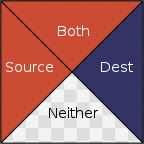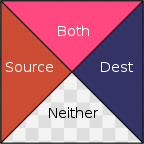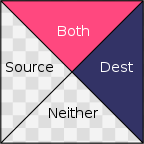## Porter/Duff Compositing and Blend Modes

In the Porter/Duff compositing algebra, images are equipped with an alpha channel that determines on a per-pixel basis whether the image is there or not. When the alpha channel is 1, the image is fully there, when it is 0, the image isn’t there at all, and when it is in between, the image is partially there. In other words, the alpha channel describes the shape of the image, it does not describe opacity. The way to think of images with an alpha channel is as irregularly shaped pieces of cardboard, not as colored glass.

Consider these two images:When we combine them, each pixel of the result can be divided into four regions:One region where only the source is present, one where only the destination is present, one where both are present, and one where neither is present.

By deciding on what happens in each of the four regions, various effects can be generated. For example, if the destination-only region is treated as blank, the source-only region is filled with the source color, and the ‘both’ region is filled with the destination color like this:The effect is as if the destination image is trimmed to match the source image, and then held up in front of it:The Porter/Duff operator that does this is called “Dest Atop”.

There are twelve of these operators, each one characterized by its behavior in the three regions: source, destination and both. The ‘neither’ region is always blank. The source and destination regions can either be blank or filled with the source or destination colors respectively.

The formula for the operators is a linear combination of the contents of the four regions, where the weights are the areas of each region:

$A_\text{src} \cdot [s] + A_\text{dest} \cdot [d] + A_\text{both} \cdot [b]$

Where $[s]$ is either 0 or the color of the source pixel, $[d]$ either 0 or the color of the destination pixel, and $[b]$ is either 0, the color of the source pixel, or the color of the destination pixel. With the alpha channel being interpreted as coverage, the areas are given by these formulas:

$A_\text{src} = \alpha_\text{s} \cdot (1 - \alpha_\text{d})$
$A_\text{dst} = \alpha_\text{d} \cdot (1 - \alpha_\text{s})$
$A_\text{both} = \alpha_\text{s} \cdot \alpha_\text{d}$

The alpha channel of the result is computed in a similar way:

$A_\text{src} \cdot [\text{as}] + A_\text{dest} \cdot [\text{ad}] + A_\text{both} \cdot [\text{ab}]$

where $[\text{as}]$ and $[\text{ad}]$ are either 0 or 1 depending on whether the source and destination regions are present, and where $[\text{ab}]$ is 0 when the ‘both’ region is blank, and 1 otherwise.

Here is a table of all the Porter/Duff operators:

 $[\text{s}]$ $[\text{d}]$ $[\text{b}]$ Src $s$ $0$ s Atop $0$ $d$ s Over $s$ $d$ s In $0$ $0$ s Out $s$ $0$ $0$ Dest $0$ $d$ d DestAtop $s$ $0$ d DestOver $s$ $d$ d DestIn $0$ $0$ d DestOut $0$ $d$ $0$ Clear $0$ $0$ $0$ Xor $s$ $d$ $0$

And here is how they look:Despite being referred to as alpha blending and despite alpha often being used to model opacity, in concept Porter/Duff is not a way to blend the source and destination shapes. It is way to overlay, combine and trim them as if they were pieces of cardboard. The only place where source and destination pixels are actually blended is along the antialiased edges.

Blending
Photoshop and the Gimp have a concept of layers which are images stacked on top of each other. In Porter/Duff, stacking images on top of each other is done with the “Over” operator, which is also what Photoshop/Gimp use by default to composite layers:Conceptually, two pieces of cardboard are held up with one in front of the other. Neither shape is trimmed, and in places where both are present, only the top layer is visible.

A layer in these programs also has an associated Blend Mode which can be used to modify what happens in places where both are visible. For example, the ‘Color Dodge’ blend mode computes a mix of source and destination according to this formula:

$\begin{equation*} B(s,d)= \begin{cases} 0 & \text{if $$d=0$$,} \\ 1 & \text{if $$d \ge (1 - s)$$,} \\ d / (1 - s) & \text{otherwise} \end{cases} \end{equation*}$

The result is this:Unlike with the regular Over operator, in this case there is a substantial chunk of the output where the result is actually a mix of the source and destination.

Layers in Photoshop and Gimp are not tailored to each other (except for layer masks, which we will ignore here), so the compositing of the layer stack is done with the source-only and destination-only region set to source and destination respectively. However, there is nothing in principle stopping us from setting the source-only and destination-only regions to blank, but keeping the blend mode in the ‘both’ region, so that tailoring could be supported alongside blending. For example, we could set the ‘source’ region to blank, the ‘destination’ region to the destination color, and the ‘both’ region to ColorDodge:Here are the four combinations that involve a ColorDodge blend mode:In this model the original twelve Porter/Duff operators can be viewed as the results of three simple blend modes:

 Source: $B(s, d) = s$ Dest: $B(s, d) = d$ Zero: $B(s, d) = 0$

In this generalization of Porter/Duff the blend mode is chosen from a large set of formulas, and each formula gives rise to four new compositing operators characterized by whether the source and destination are blank or contain the corresponding pixel color.

Here is a table of the operators that are generated by various blend modes:The general formula is still an area weighted average:

$A_\text{src} \cdot [s] + A_\text{dest} \cdot [d] + A_\text{both}\cdot B(s, d)$

where [s] and [d] are the source and destination colors respectively or 0, but where $B(s, d)$ is no longer restricted to one of $0$, $s$, and $d$, but can instead be chosen from a large set of formulas.

The output of the alpha channel is the same as before:

$A_\text{src} \cdot [\text{as}] + A_\text{dest} \cdot [\text{ad}] + A_\text{both} \cdot [\text{ab}]$

except that [ab] is now determined by the blend mode. For the Zero blend mode there is no coverage in the both region, so [ab] is 0; for most others, there is full coverage, so [ab] is 1.

## Big-O Misconceptions

In computer science and sometimes mathematics, big-O notation is used to talk about how quickly a function grows while disregarding multiplicative and additive constants. When classifying algorithms, big-O notation is useful because it lets us abstract away the differences between real computers as just multiplicative and additive constants.

Big-O is not a difficult concept at all, but it seems to be common even for people who should know better to misunderstand some aspects of it. The following is a list of misconceptions that I have seen in the wild.

But first a definition: We write

$f(n) = O(g(n))$

when $f(n) \le M g(n)$ for sufficiently large $n$, for some positive constant $M$.

Misconception 1: “The Equals Sign Means Equality”

$f(n) = O(g(n))$

is a widespread travestry. If you take it at face value, you can deduce that since $5 n$ and $3 n$ are both equal to $O(n)$, then $3 n$ must be equal to $5 n$ and so $3 = 5$.

The expression $f(n) = O(g(n))$ doesn’t type check. The left-hand-side is a function, the right-hand-side is a … what, exactly? There is no help to be found in the definition. It just says “we write” without concerning itself with the fact that what “we write” is total nonsense.

The way to interpret the right-hand side is as a set of functions:

$O(f) = \{ g \mid g(n) \le M f(n) \text{ for some $$M > 0$$ for large $$n$$}\}.$

With this definition, the world makes sense again: If $f(n) = 3 n$ and $g(n) = 5 n$, then $f \in O(n)$ and $g \in O(n)$, but there is no equality involved so we can’t make bogus deductions like $3=5$. We can however make the correct observation that $O(n) \subseteq O(n \log n)\subseteq O(n^2) \subseteq O(n^3)$, something that would be difficult to express with the equals sign.

Misconception 2: “Informally, Big-O Means ‘Approximately Equal’"

If an algorithm takes $5 n^2$ seconds to complete, that algorithm is $O(n^2)$ because for the constant $M=7$ and sufficiently large $n$, $5 n^2 \le 7 n^2$. But an algorithm that runs in constant time, say 3 seconds, is also $O(n^2)$ because for sufficiently large $n$, $3 \le n^2$.

So informally, big-O means approximately less than or equal, not approximately equal.

If someone says “Topological Sort, like other sorting algorithms, is $O(n \log n)$", then that is technically correct, but severely misleading, because Toplogical Sort is also $O(n)$ which is a subset of $O(n \log n)$. Chances are whoever said it meant something false.

If someone says “In the worst case, any comparison based sorting algorithm must make $O(n \log n)$ comparisons” that is not a correct statement. Translated into English it becomes:

“In the worst case, any comparison based sorting algorithm must make fewer than or equal to $M n \log (n)$ comparisons”

which is not true: You can easily come up with a comparison based sorting algorithm that makes more comparisons in the worst case.

To be precise about these things we have other types of notation at our disposal. Informally:

 $O()$: Less than or equal, disregarding constants $\Omega()$: Greater than or equal, disregarding constants $o()$: Stricly less than, disregarding constants $\Theta()$: Equal to, disregarding constants

and some more. The correct statement about lower bounds is this: “In the worst case, any comparison based sorting algorithm must make $\Omega(n \log n)$ comparisons”. In English that becomes:

“In the worst case, any comparison based sorting algorithm must make at least $M n \log (n)$ comparisons”

which is true. And a correct, non-misleading statement about Topological Sort is that it is $\Theta(n)$, because it has a lower bound of $\Omega(n)$ and an upper bound of $O(n)$.

Misconception 3: “Big-O is a Statement About Time”

Big-O is used for making statements about functions. The functions can measure time or space or cache misses or rabbits on an island or anything or nothing. Big-O notation doesn’t care.

In fact, when used for algorithms, big-O is almost never about time. It is about primitive operations.

When someone says that the time complexity of MergeSort is $O(n \log n)$, they usually mean that the number of comparisons that MergeSort makes is $O(n \log n)$. That in itself doesn’t tell us what the time complexity of any particular MergeSort might be because that would depend how much time it takes to make a comparison. In other words, the $O(n \log n)$ refers to comparisons as the primitive operation.

The important point here is that when big-O is applied to algorithms, there is always an underlying model of computation. The claim that the time complexity of MergeSort is $O(n \log n)$, is implicitly referencing an model of computation where a comparison takes constant time and everything else is free.

Which is fine as far as it goes. It lets us compare MergeSort to other comparison based sorts, such as QuickSort or ShellSort or BubbleSort, and in many real situations, comparing two sort keys really does take constant time.

However, it doesn’t allow us to compare MergeSort to RadixSort because RadixSort is not comparison based. It simply doesn’t ever make a comparison between two keys, so its time complexity in the comparison model is 0. The statement that RadixSort is $O(n)$ implicitly references a model in which the keys can be lexicographically picked apart in constant time. Which is also fine, because in many real situations, you actually can do that.

To compare RadixSort to MergeSort, we must first define a shared model of computation. If we are sorting strings that are $k$ bytes long, we might take “read a byte” as a primitive operation that takes constant time with everything else being free.

In this model, MergeSort makes $O(n \log n)$ string comparisons each of which makes $O(k)$ byte comparisons, so the time complexity is $O(k\cdot n \log n)$. One common implementation of RadixSort will make $k$ passes over the $n$ strings with each pass reading one byte, and so has time complexity $O(n k)$.

Misconception 4: Big-O Is About Worst Case

Big-O is often used to make statements about functions that measure the worst case behavior of an algorithm, but big-O notation doesn’t imply anything of the sort.

If someone is talking about the randomized QuickSort and says that it is $O(n \log n)$, they presumably mean that its expected running time is $O(n \log n)$. If they say that QuickSort is $O(n^2)$ they are probably talking about its worst case complexity. Both statements can be considered true depending on what type of running time the functions involved are measuring.

## Sysprof 1.2.0

A new stable release of Sysprof is now available. Download version 1.2.0.